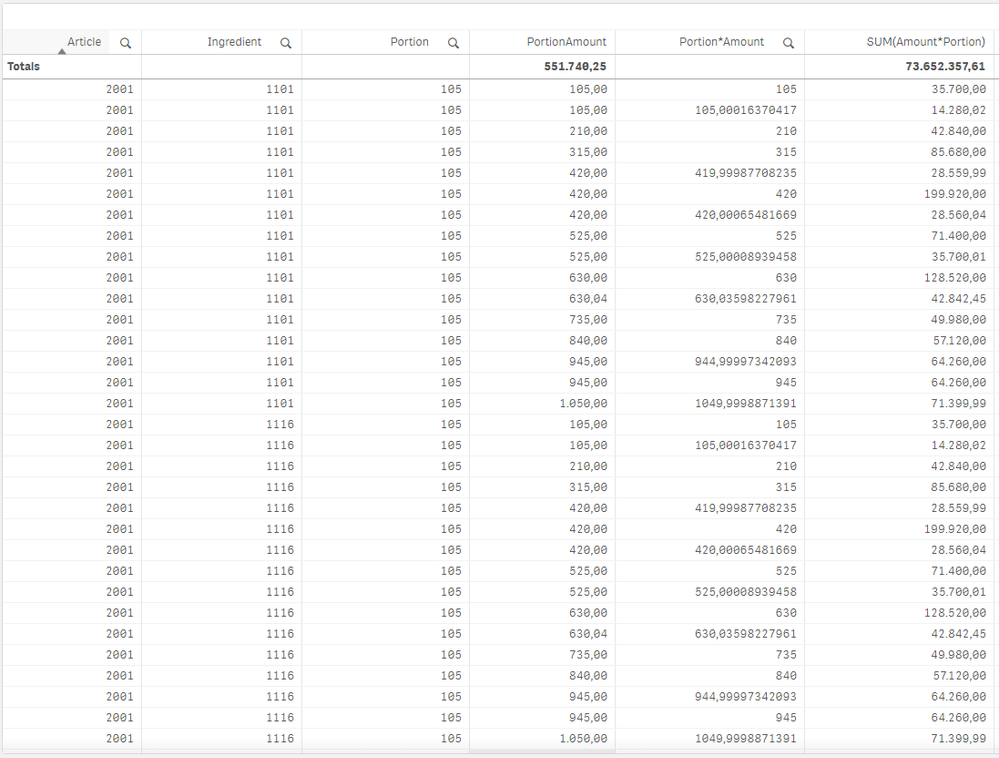# App Development

Announcements
Now Live: Qlik Sense SaaS Simplified Authoring – Analytics Creation for Everyone: READ DETAILS
cancel
Showing results for
Did you mean:Contributor III

## Aggregation pivot calculation

Good morning,

I've this table below. The portion refers to the batch amount per article. If I multiply the portion by the amount per portion produced, I get the overall amount (dimension Portion*Amount). The PortionAmount is a master measure with the same formula (with the benefit that I can add an aggregation function from the list). I can't use this master measure in my pivot table (on another sheet). If I aggregate this with SUM, the total is very different. The total amount in "PortionAmount", the master measure is the right (total) amount. Why are these two totals different?

How can I get an aggregation with the right total amount, which works in a pivot table as a measure?TableMaster measure

Labels (3)

• ### Pivot Measure

3 RepliesMVP

hi, it looks like each table row has manny records associated, 35700/105 is 340, so 340 rows with the same numbers are counted in the sum.

This is probably an issue in how is created the data model (just probably).

To get the expected total maybe with Sum(Aggr(Portion*Amount, Article, Ingredient, Portion))Contributor III
Author

Thanks for the tip, it was indeed that portion (batch size) should not be summed. But the formula didn't work. I found the function 'Only', which is an aggregation function. I'm now at: SUM(Amount)*Only(Portion). It don't add up if I remove the Portion dimension because of the Only function. How can I turn this Only function in my Sum function to fix this problem?MVP

Have you tried the expression I post before?:

Sum(Aggr(Portion*Amount, Article, Ingredient, Portion))

or:

Sum(Aggr(Sum(Portion)*Amount, Article, Ingredient, Portion))Tags